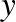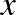$$\newcommand{\id}{\mathrm{id}}$$ $$\newcommand{\Span}{\mathrm{span}}$$ $$\newcommand{\kernel}{\mathrm{null}\,}$$ $$\newcommand{\range}{\mathrm{range}\,}$$ $$\newcommand{\RealPart}{\mathrm{Re}}$$ $$\newcommand{\ImaginaryPart}{\mathrm{Im}}$$ $$\newcommand{\Argument}{\mathrm{Arg}}$$ $$\newcommand{\norm}{\| #1 \|}$$ $$\newcommand{\inner}{\langle #1, #2 \rangle}$$ $$\newcommand{\Span}{\mathrm{span}}$$

5.E: Eigenvalue Problems (Exercises)

$$\newcommand{\vecs}{\overset { \rightharpoonup} {\mathbf{#1}} }$$

$$\newcommand{\vecd}{\overset{-\!-\!\rightharpoonup}{\vphantom{a}\smash {#1}}}$$

These are homework exercises to accompany Libl's "Differential Equations for Engineering" Textmap. This is a textbook targeted for a one semester first course on differential equations, aimed at engineering students. Prerequisite for the course is the basic calculus sequence.

5.1: Sturm-Liouville problems

Exercise 5.1.4: Find eigenvalues and eigenfunctions of

$y''+ \lambda y=0,~~~y(0)-y'(0)=0,~~~y(1)=0.$

Exercise 5.1.5: Expand the function $$f(x)=x$$ on $$0 \leq x \leq 1$$ using the eigenfunctions of the system

$y''+ \lambda y=0,~~~y'(0)=0,~~~y(1)=0.$

Exercise 5.1.6: Suppose that you had a Sturm-Liouville problem on the interval $$[0,1]$$ and came up with $$y_n(x)=\sin(\gamma nx)$$, where $$\gamma >0$$ is some constant. Decompose $$f(x)=x, 0<x<1$$, in terms of these eigenfunctions.

Exercise 5.1.7: Find eigenvalues and eigenfunctions of

$y'^{(4)}+ \lambda y=0,~~~y(0)=0,~~~y'(0)=0,~~~y(1)=0~~~y'(1)=0.$

This problem is not a Sturm-Liouville problem, but the idea is the same.

Exercise 5.1.8 (more challenging): Find eigenvalues and eigenfunctions for

$\frac{d}{dx}(e^xy')+ \lambda e^xy=0,~~~y(0)=0,~~~y(1)=0.$

Hint: First write the system as a constant coeﬃcient system to ﬁnd general solutions. Do note that Theorem 5.1.1 guarantees $$\lambda \geq 0$$.

Exercise 5.1.101: Find eigenvalues and eigenfunctions of

$y''+ \lambda y=0,~~~y(-1)=0,~~~y(1)=0.$

Exercise 5.1.102: Put the following problems into the standard form for Sturm-Liouville problems, that is, ﬁnd $$p(x),q(x), r(x), \alpha_1,\alpha_,\beta_1,\beta_1,$$, and decide if the problems are regular or not.

$$a) ~xy''+\lambda y=0$$ for $$0<x<1,y(0)=0, y(1)=0,$$

$$b) ~ (1+x^2)y''+2xy'+(\lambda -x^2)y=0$$ for $$-1<x<1,y(-1)=0,y(1)+y'(1)=0$$

5.2: Application of eigenfunction series

Exercise 5.2.2: Suppose you have a beam of length $$5$$ with free ends. Let $$y$$ be the transverse deviation of the beam at position $$x$$ on the beam $$(0<x<5)$$. You know that the constants are such that this satisﬁes the equation $$y_{tt}+4y_{xxxx}=0$$. Suppose you know that the initial shape of the beam is the graph of $$x(5-x)$$, and the initial velocity is uniformly equal to $$2$$ (same for each $$x$$) in the positive $$y$$ direction. Set up the equation together with the boundary and initial conditions. Just set up, do not solve.

Exercise 5.2.3: Suppose you have a beam of length $$5$$ with one end free and one end ﬁxed (the ﬁxed end is at $$x=5$$). Let $$u$$ be the longitudinal deviation of the beam at position $$x$$ on the beam $$(0<x<5)$$. You know that the constants are such that this satisﬁes the equation $$u_{tt}=4u_{xx}$$. Suppose you know that the initial displacement of the beam is $$\frac{x-5}{50}$$, and the initial velocity is $$\frac{-(x-5)}{100}$$ in the positive $$u$$ direction. Set up the equation together with the boundary and initial conditions. Just set up, do not solve.

Exercise 5.2.4: Suppose the beam is $$L$$ units long, everything else kept the same as in (5.2.2). What is the equation and the series solution?

Exercise 5.2.5: Suppose you have

$a^4y_{xxxx}+y_{tt}=0~~~~(0<x<1,t>0), \\ y(0,t)=y_{xx}(0,t)=0, \\ y(1,t)=y_{xx}(1,t)=0, \\ y(x,0)=f(x),~~~~y_t(x,0)=g(x).$

That is, you have also an initial velocity. Find a series solution. Hint: Use the same idea as we did for the wave equation.

Exercise 5.2.101: Suppose you have a beam of length $$1$$ with hinged ends. Let $$y$$ be the transverse deviation of the beam at position $$x$$ on the beam ($$0<x<1$$). You know that the constants are such that this satisﬁes the equation $$y_{tt}+4y_{xxxx}=0$$. Suppose you know that the initial shape of the beam is the graph of $$\sin(\pi x)$$, and the initial velocity is $$0$$. Solve for $$y$$.

Exercise 5.2.102: Suppose you have a beam of length $$10$$ with two ﬁxed ends. Letbe the transverse deviation of the beam at positionon the beam ($$0<x<10$$). You know that the constants are such that this satisﬁes the equation $$y_{tt}+9y_{xxxx}=0$$. Suppose you know that the initial shape of the beam is the graph of $$\sin(\pi x)$$, and the initial velocity is uniformly equal to $$x(10-x)$$. Set up the equation together with the boundary and initial conditions. Just set up, do not solve.

5.3: Steady periodic solutions

Exercise 5.3.5: Suppose that the forcing function for the vibrating string is $$F_0 \sin(\omega t)$$. Derive the particular solution $$y_p$$.

Exercise 5.3.6: Take the forced vibrating string. Suppose that $$L=1,a=1$$. Suppose that the forcing function is the square wave that is $$1$$ on the interval $$0<x<1$$ and $$-1$$on the interval $$-1<x<0$$. Find the particular solution. Hint: You may want to use result of Exercise 5.3.5.

Exercise 5.3.7: The units are cgs (centimeters-grams-seconds). For $$k=0.005, \omega =1.991 \times 10^{-7},A_0=20$$. Find the depth at which the temperature variation is half ($$\pm 10$$ degrees) of what it is on the surface.

Exercise 5.3.8: Derive the solution for underground temperature oscillation without assuming that $$T_0=0$$.

Exercise 5.3.101: Take the forced vibrating string. Suppose that $$L=1,a=1$$. Suppose that the forcing function is a sawtooth, that is $$|x|-\frac{1}{2}$$ on $$-1<x<1$$ extended periodically. Find the particular solution.

Exercise 5.3.102: The units are cgs (centimeters-grams-seconds). For $$k=0.01, \omega =1.991 \times 10^{-7},A_0=25$$. Find the depth at which the summer is again the hottest point.